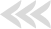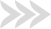#####GET QUOTE IN 2 MINUTES*## Computer Graphics Quiz

### CallTutors Guarantees

• Lowest Price Guaranteed
• Plagiarism Free Guaranteed
• 24 * 7 Availability
• Native Experienced Experts
• Free Revisions### Price Includes

Turnitin Report

\$20.99
free

Limitless Amendments

\$31.49
free

Bibliography

\$17.05
free

Outline

\$6.55
free

Title Page

\$6.55
free

Formatting

\$10.49
free

Get all these features

\$93.12
free

CS 4406: COMPUTER GRAPHICS

True/False: The 3D Cartesian coordinate space is divided into 6 quadrants.

Select one:

True

False

Question
2

True/False: In 2D Cartesian coordinates, any two lines that are not parallel will meet in a point.

Select one:

True

False

Question
3

What color does the following hexadecimal number 0x000000 specify?

Select one:

1. White
2. Black
3. Yellow
4. Red

Question
4

True/False: Of the three colors in the RGB model, blue has the brightest luminance.

Select one:

True

False

Question
5

The transparency for a color is called the _____ and its value is a number between 0.0 and 1.0.

Select one:

1. Alpha Level
2. color gamut
3. Mach bands
4. emissive colorThe correct answer is: Alpha Level

Question
6

The range of colors that a display device can represent is called the:

Select one:

1. Color gamut
2. mach bands
3. the CMYK of the device

The correct answer is: Color gamut

Question
7

True/False: In an object with an alpha level of 1.0, you can see the objects that behind it?

Select one:

True

False

Question
8

True/False: The difference between WebGL and OpenGL is that WebGL only supports 2D graphics and OpenGL supports both 2D and 3D graphics.

Select one:

True

False

Question
9

The position that you are viewing a scene from is called the:

Select one:

1. eye point
2. view reference point
3. viewport
4. view frustum

The correct answer is: eye point

Question
10

True/False: An orthographic projection, projects all of the objects in the scene to the viewing plane by parallel lines.

Select one:

True

False

Question
11

Which projection do the following objects represent?

Select one:

1. Parallel projection
2. Orthographic projection
3. Perspective projection
4. Scaled projection

The correct answer is: Perspective projection

Question
12

True/False: Clipping defines parts of the scene that you do not want to display.

Select one:

True

False

Question
13

Modeling an object about its local origin involves defining it in terms of:

Select one:

1. modeling coordinates
2. eye coordinates
3. euclidean coordinates
4. modeling transformations

The correct answer is: modeling coordinates

Question
14

A vertex in graphics modeling defines a:

Select one:

1. Point
2. Line
3. Polygon
4. None of these responses

Question
15

Which of the following shows obvious aliasing artifacts?

Select one:

1. line a
2. line b
3. line c
4. line d

The correct answer is: line b

Question
16

Which of the following is an antialiasing method?

Select one:

1. pixel supersampling
2. geometry sequencing
3. unisample antialiasing
4. spatial aliasing

The correct answer is: pixel supersampling

Question
17

What geometry type will the following pseudo code generate?

N = 12

set vertex at (1,0,90)

for i = 0 to N

set vertex at (1, 360/I, 90-180/N)

Select one:

1. Triangle Fan
2. Triangle Strip

The correct answer is: Triangle Fan

Question
18

True/False: A transformation is a function that takes geometry and produces new geometry.

Select one:

True

False

Question
19

True/False: To rotate geometry 90 degrees you would use the translation form of transformation?

Select one:

True

False

Question
20

Which of the following types of transformations should be used to transform the picture on the left into the picture on the right?

Select one:

1. Rotation
2. Translation
3. Rendering

Question
21

True/False: Transformations are mathematical operations that map 3D space to 2D space and so mathematics has standard ways to represent them.

Select one:

True

False

Question
22

True/False: In Three.js, the CylinderGeometry object is created from a series of vertices?

Select one:

True

False